## Saturday, October 31, 2009

### CapacitorsCapacitors are devices used to store electric charge and usually involve conducting plates separated by an insulator. (One plate is positive while the other is negative.)

When the capacitor is connected to a battery, electrons flow from the negative terminal to plate

Capacitors are widely used in electronic circuits to block the flow of direct current (D.C) while allowing alternating current(A.C) to pass, to filter out interference, to smooth the output of power supplies, and for many other purposes.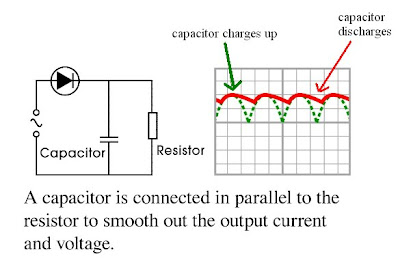SPM past year questions:
D__Desktop_r

related video from http://ec.europa.eu/research/mariecurieactions/

## Thursday, October 29, 2009

### Physics Definition

Normally this is test in paper 2 and 3, remember to answer in full sentence DO NOT write equation !!!
example :
a. ) momentum is product of mass and velocity ( correct )
b. ) momentum = mass x velocity ( wrong)
SPM Physics Terms and Definition

## Saturday, October 24, 2009

### How to answer SPM physics (paper 2 and 3)?

Semua maklumat yang diberi adalah dari JUJ Pahang 2009.

1. How to write experiment precaution ?

prec

2. Tips to answer paper 2

p2

3. Tips to answer paper 3

D Desktop g3

## Friday, October 23, 2009

### Tips for paper 2 ( SPM physics)

1. Definition of the term in Physics
Force, work, inertia, impulsive force, coherent...

2. The principle in Physics and applications
Force in equilibrium, thermal equilibrium, Pascal's principle, conservation of momentum.

3. The Law in Physics and application
Hooke's Law, Ohm's Law, Boyle's Law, Charles Law

4. Graph skills
Axis, plotting, scale, straight or curve line, gradient, extrapolation.

5. Drawing skills
Total internal reflection, lens ray, inteferens, field circuit, refraction of wave

Mini quizzes :
1. What is meant by frequency of oscillation on spring?
2. What is meant by pressure ?
3. Define speed.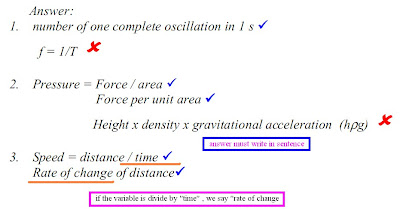4.

## Thursday, October 22, 2009

### How to answer paper 1 (SPM Physics)

SPM at the corner already , it time to refresh some common exam tips. One way
1. Cancel or mark (x) which is wrong option then choose.
2. Always refer to the formula given on page 2
3. Blacken each finished answer immediately.
Example:
1.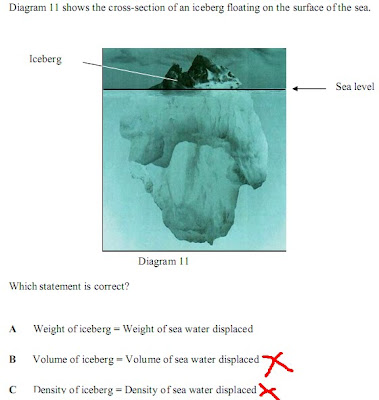2.### Displacement- time graph

What to learn in this unit?
1. plot and interpret displacement-time graphs.
2. determine distance, displacement and velocity from a displacement-time graph
3. deduce information from the shape of a displacement-time graph

Content :
On a displacement-time graph, the gradient of the graph is numerically equal to the velocity of the object.

a.On a displacement-time graph, a horizontal line (gradient = ________ ) shows that an object s stationary.Object moving with constant velocity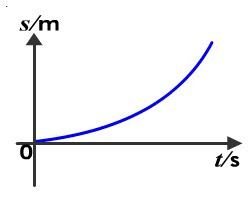Object moving with increasing velocity.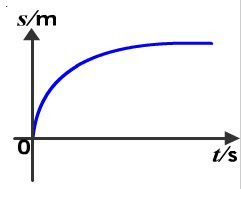Object moving with decreasing velocity.

## Tuesday, October 20, 2009

### The Equation of linear motion

The equations of linear motion is apply to bodies moving linearly with uniform acceleration .
There are 5 variables in the equation
v = final velocity,
u
= initial velocity,
s = displacement,
a = acceleration,
t
= time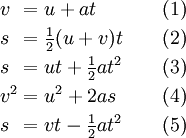You should be able to solve problems using equation about.

Example :
1. A cyclist accelerates uniformly at 1.2 ms-2 in 10 s from rest. What is his displacement at this time?

Tips:
• Rest mean zero velocity !!
• You need to know 3 variables to find out the required quantities .
Suggested solutionGiven;

u = 0 ms-1
a = 1.2 ms-2
t = 10 s
v = ? ( since v is not given you are unable to calculate s using equation 1, 2, 4 and 5)

substitute all given information into equation 3
s= ut+ 1/2 at
2
s= (0) (10) + 1/2 (1.2) (10)2
=60 m (very often, student forget to mention the unit. )

2. A car approaches a traffic light at 15m/s. The traffic light turns red and the driver applies the brakes at the distance of 25 m from the junction and decelerate at 5 ms-2. Can the car come to complete stop before reaching the stop line at the traffic light? (ans : yes)

Tips:
• remember to insert the negative sign (-) for decelerate

## Friday, October 16, 2009

### Last minutes SPM physcis check list

5UM and 5 UKM students pls download.
Fill in the blank i will discuss with you all after 2nd trial.
All the best ...good luck in your coming SPM

tip jawab

### Acceleration and deceleration

Dear our form 4 students answer following question before star your lesson.

Pretest:

1. state the meaning and unit for the symbol
i) a
ii) v
iii) u
iv) t

2. Define acceleration and deceleration

Lesson start:

Acceleration is the rate of change of velocity. ( 1 mark in paper 2 )

An object accelerates when it moves with increasing velocity.

An object decelerates when is getting slower, its velocity is decreasing.

Equation :where

a: acceleration ( ms-2)

u: initial velocity ( ms-1)

v: final velocity ( ms-1)

t: time ( second)

Calculation:

Tips:

First of all find out all useful info as highlighted. Then substitute the info given in equation, while you are calculating check whether need to change units or not. (e.g., from km to m, hour to seconds)

email your solution to fizikf5@gmail.com

1.An athletic runs from the starting line and achieves a velocity of 12 m/s in 3 s. Find his/her acceleration. (ans: 4 ms-2)

2. A track travels along a road with a velocity of 20 m/s the driver slam on the brake when a chicken suddenly runs across the road. Assuming the truck take 8s to complete stop from the time the driver hit the brake, determine the acceleration of the lorry. (ans: -2.5 ms-2)

3. A motorcycle move with a velocity of 25 m/s. the motorcyclist brakes and its velocity decreases uniformly to 10 m/s in 3s. The motorcycle continues to slow down with the same acceleration until it comes to a stop. Find the time taken for the motorcycle to stop. (ans: 5s)

* Step 1 : Determine its acceleration in the first 3s,

Step 2 : Determine the time taken .

For more detail study the graph below.